# 31. IVIM¶

## 31.1. Basics of IVIM¶

Intravoxel incoherent motion (IVIM) imaging is a type of diffusion-weighted (DW) MRI that separates the contributions of molecular diffusion and microcirculation (microperfusion) to the diffusion-weighted signal [LeBihan1988].

Diffusion-weighted MRI (DWI) data at different b-values, S(b), are described by the model expression with separate terms due to microcirculation and diffusion (31.1):

(31.1)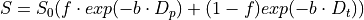Here S0=S(b=0) is the unweighted signal, Dp is the perfusion-dominated pseudodiffusion coefficient (denoted D* in some sources), Dt is the true diffusion coefficient (or D), and f is the perfusion fraction (also referred to as microcirculation fraction) [Koh2011], [LeBihan2019].

In FireVoxel, IVIM modeling is available via Dynamic Analysis > Calculate Parametric Map, Models 17 and 18, which represent two different computational approaches to the analysis of multi-b-value DWI data.

Aside from the choice of the models, the processing routine (which includes loading and preparing data, setting up the model analysis, and output options) is similar to the routine for Diffusion-Weighted Imaging (DWI).Fig. 31.1 IVIM model fit (blue - data, red - fit, orange - monexponential fit to b>200 s/mm2).

## 31.2. Model 17: IVIM bi-exponential diffusion Segmented Fit¶

Model 17 implements the segmented curve fit, as proposed by Sigmund et al [Sigmund2011] for analyzing IVIM data in breast lesions. This fit is performed in two steps (Fig. 31.1). First, the data at higher b-values (b>~200 s/mm2) are used to compute the true diffusion coefficient Dt and perfusion fraction f. These values are then fixed and used to determine Dp.

Model 17 requires DW data with at least three b-values greater than 200 s/mm2. If the user attempts to process an incompatible dataset, the model shows an error message, advising the user to select Model 18: IVIM bi-exponential fit.

This goal of this approach is to improve the robustness of the model output parameters by reducing the number of parameters at each fitting step. This approach may be preferable for datasets with relatively few b-values and signal data with higher noise, such as when performing model analysis on a voxel-by-voxel basis.

The pseudodiffusion coefficient Dp is typically much greater than Dt. Therefore for b much greater than ~1/Dp (e.g., for Dp~10 x 10-3 mm2/s, b>100 s/mm2), the contribution of the pseudodiffusion term to the signal S(b) is small compared to the contribution of the true diffusion form. Considering only these higher b-values, the IVIM model equation reduces to a monoexponential fit expression with only f and Dt:

(31.2)Using this monoexponential fit, Dt can be determined from data at b-values above a given threshold (in FireVoxel, b>200 s/mm2 by default), with zero intercept Si and S0=S(b=0) used to determine the perfusion fraction f:

(31.3)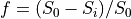With Dt and f fixed at these values, the values of Dp can be determined by using a nonlinear fit of the entire dataset with (eq).

The parameters of model 17 are as follows (Fig. 31.2):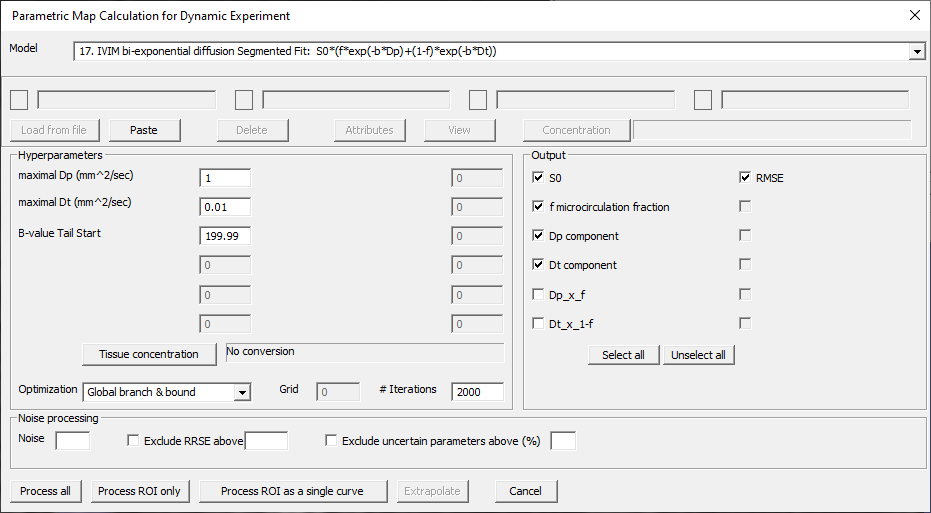Fig. 31.2 Model 17 IVIM dialog with default hyperparameters.

Model 17 hyperparameters:

• maximal Dp (mm^/sec) - Upper limit on pseudodiffusion coefficient Dp. Default: 1 (mm2/s).

• maximal Dt (mm^/sec) - Upper limit on true diffusion coefficient Dt. Default: 0.01 (mm2/s).

• B-value Tail Start - The data at b-values higher than this threshold value are used to determine Dt and f. Default: 199.99 (s/mm2). At least three b-values greater than this threshold are required. If this condition is not met, an error message is shown. Model 18, which does not have this restriction, can be used instead.

Model 17 output parameters:

• S0 - Unweighted signal S0=S(b=0)

• f microcirculation fraction - Microcirculation (microperfusion) fraction f (unitless)

• Dp component - pseudodiffusion coefficient Dp (mm2/s)

• Dt component - true diffusion coefficient Dt (mm2/s)

• Dp x f - Composite parameter describing microcirculation (microperfusion) (mm2/s)

• Dt x 1-f - Composite parameter describing true diffusion (mm2/s)

• RMSE - Root-mean-square error of the model fit

The output of Model 17 with Process ROI as a single curve option yields fitted parameters that can be exported (Fig. 31.3).

## 31.3. Model 18: IVIM bi-exponential diffusion¶

Model 18 performs regular nonlinear curve fit of all signal intensities at all b-values. This model requires a 4D DW dataset consisting of 3D volumes acquired at different b-values. There is no restriction on the number of b-values. However, analysis of datasets with few b-values (n~5) is likely to yield fitted parameters with larger uncertainties than analysis of larger datasets. This may also entail convergence of the fit to various local minima depending on the values of hyperparameters.

The parameters of Model 18 are as follows (Fig. 31.4).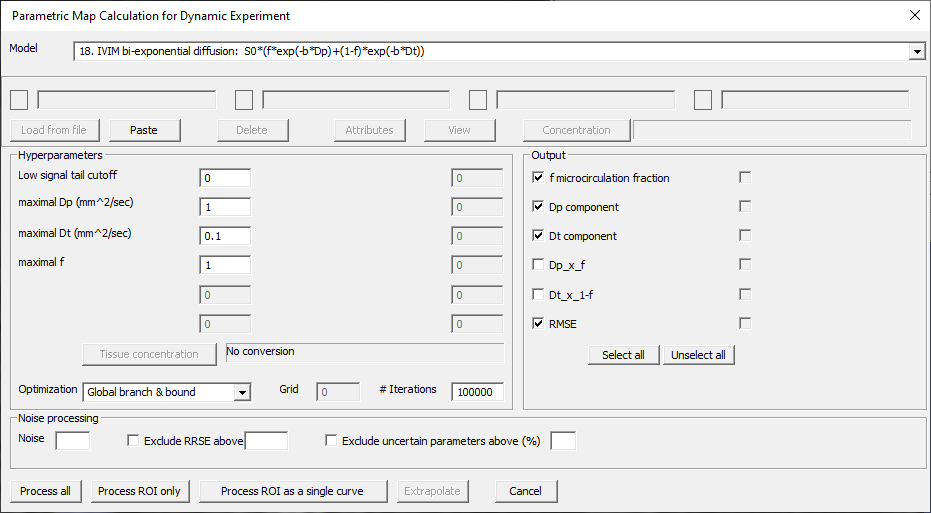Fig. 31.4 Model 18 IVIM (regular) biexponential fit dialog.

Model 18 hyperparameters:

• Low signal tail cutoff - Signal value below which data are excluded from fit. Default: 0.

• maximal Dp (mm^/sec) - Upper limit on pseudodiffusion coefficient Dp. Default: 1 (mm2/s).

• maximal Dt (mm^/sec) - Upper limit on true diffusion coefficient Dt. Default: 0.01 (mm2/s).

• maximal f - Upper limit of microcirculation fraction f. Default: 1.

Model 18 parameters:

• f microcirculation fraction - Microcirculation (microperfusion) fraction f (unitless)

• Dp component - pseudodiffusion coefficient Dp (mm2/s)

• Dt component - true diffusion coefficient Dt (mm2/s)

• Dp x f - Composite parameter describing microcirculation (microperfusion) (mm2/s)

• Dt x 1-f - Composite parameter describing true diffusion (mm2/s)

• RMSE - Root-mean-square error of the model fit

The results of Model 18 (Fig. 31.5) for the same data as in Fig. 31.3 enables comparisons between the two fitting approaches.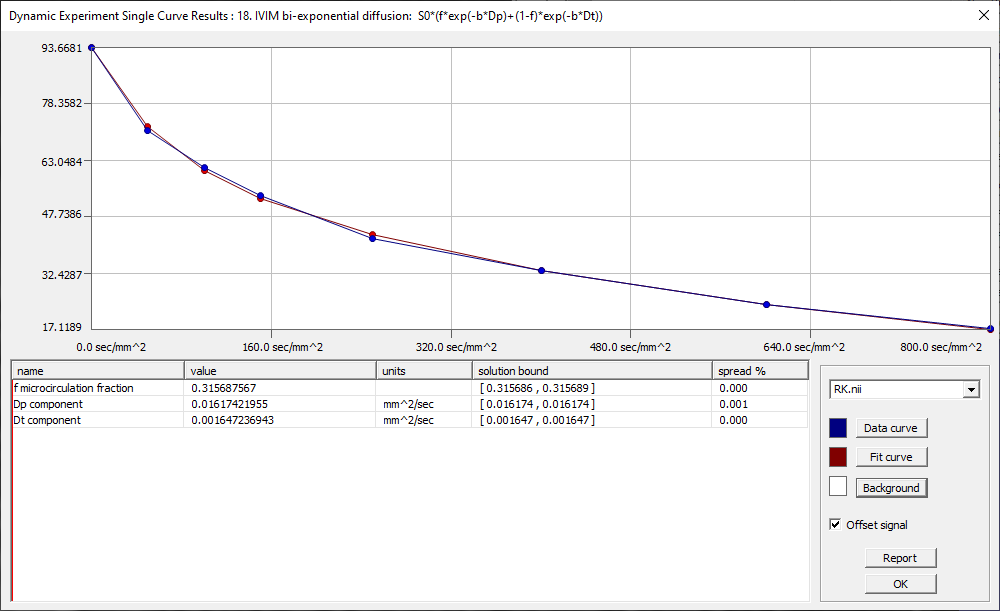Fig. 31.5 Model 18 IVIM (regular) biexponential output.

LeBihan1988

Le Bihan D, Breton E, Lallemand D, Aubin ML, Vignaud J, Laval-Jeantet M. Separation of diffusion and perfusion in intravoxel incoherent motion MR imaging. Radiology. 1988;168(2):497-505. doi: 10.1148/radiology.168.2.3393671. PMID: 3393671

LeBihan2019

Le Bihan D. What can we see with IVIM MRI? Radiology. Neuroimage. 2019;187:56-67. doi: 10.1016/j.neuroimage.2017.12.062. PMID: 29277647

Koh2011

Koh DM, Collins DJ, Orton MR. Intravoxel incoherent motion in body diffusion-weighted MRI: reality and challenges. AJR Am J Roentgenol. 2011;196(6):1351-61. doi: 10.2214/AJR.10.5515. PMID: 21606299

Sigmund2011

Sigmund EE, Cho GY, Kim S, Finn M, Moccaldi M, Jensen JH, Sodickson DK, Goldberg JD, Formenti S, Moy L. Intravoxel incoherent motion imaging of tumor microenvironment in locally advanced breast cancer. Magn Reson Med. 2011; 65(5):1437-47. doi: 10.1002/mrm.22740. Epub 2011 Feb 1. PMID: 21287591